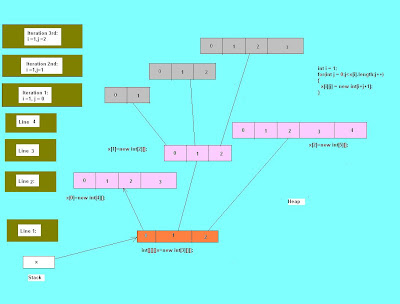## Friday, February 1, 2008

### 3 D Arrays:3 D Arrays:

Today I will explain how 3 D arrays are created on the heap,Lets consider following example while demonstrating:
public class test{
public stati void main(String[] arg){
int[][][]x=new int[][]; // Line 1
int i = 1,j;
x=new int[]; //Line 2
x=new int[]; // Line 3
x=new int[]; //Line 4

for(j=0;j{
x[i][j]=new int[i+j+1];
}
}
}